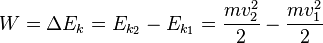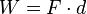# Work (physics) facts for kids

Kids Encyclopedia Facts

In physics, a force does work when it acts on a body and there is a displacement of the point of application in the direction of the force.

The work done by a force acting on a body is the force along the direction of the displacement multiplied by the displacement of the point of application.

It is the force that does the work, not the agent that created the force. Motion is a requirement of work.

Like energy, it is a scalar quantity, with SI units of joules. Heat conduction is not considered to be a form of work, since there is no macroscopically measurable force, only microscopic forces occurring in atomic collisions. The term work was created in the 1830s by the French mathematician Gaspard-Gustave Coriolis.

According to the work-energy theorem if an external force acts upon a rigid object, causing its kinetic energy to change from Ek1 to Ek2, then the mechanical work (W) is given by:$W = \Delta E_k = E_{k_2} - E_{k_1} = \frac{mv_2^2}{2} - \frac{mv_1^2}{2}$

where m is the mass of the object and v is the object's velocity.

If a constant force F acts on an object while the object is displaced a distance d, and the force and displacement are parallel to each other, the work done on the object is the product of F and d:$W = F \cdot d$

If the force and the displacement are in the same direction, the work is positive. If the force and the displacement are in opposite directions the work is negative. For example, the work done by the weight on a book being lifted is negative. This is because the downward weight is in the opposite direction to the upward displacement.

## Images for kidsWork (physics) Facts for Kids. Kiddle Encyclopedia.